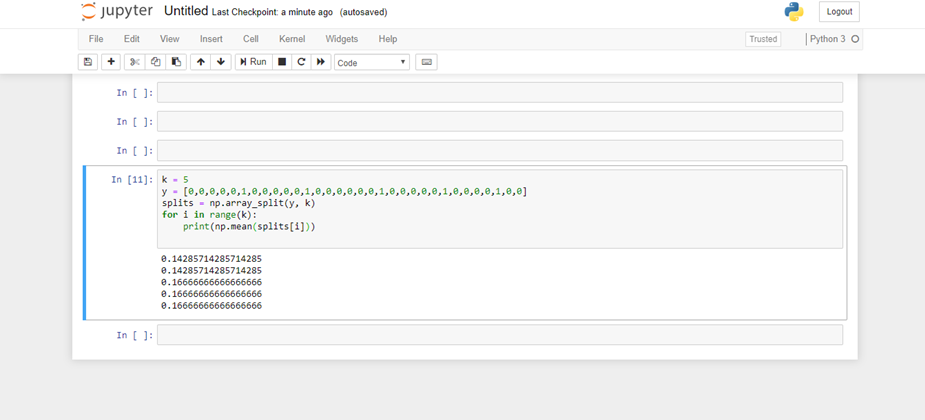1 view

I have data with 4 classes and I am trying to build a classifier. I have ~1000 vectors for one class, ~10^4 for another, ~10^5 for the third and ~10^6 for the fourth. I was hoping to use cross-validation so I looked at the scikit-learn docs .

My first try was to use StratifiedShuffleSplit but this gives the same percentage for each class, leaving the classes drastically imbalanced still.

Is there a way to do cross-validation but with the classes balanced in the training and test set?

As a side note, I couldn't work out the difference between StratifiedShuffleSplit and StratifiedKFold . The descriptions look very similar to me.

by (6.8k points)

K-Fold CV works by randomly partitioning your data into k (fairly) equal partitions. If your data were evenly balanced across classes like [0,1,0,1,0,1,0,1,0,1], randomly sampling with (or without replacement) will give you approximately equal sample sizes of 0 and 1.

However, if your data is more like[0,0,0,0,0,1,0,0,0,0,0,1,0,0,0,0,0,0,1,0,0,0,0,0,1,0,0,0,0,1,0,0] where one class over represents the data, k-fold cv without weighted sampling would give you erroneous results.

If you use ordinary k-fold CV without adjusting sampling weights from uniform sampling, then you'd obtain something like

k = 5

y = [0,0,0,0,0,1,0,0,0,0,0,1,0,0,0,0,0,0,1,0,0,0,0,0,1,0,0,0,0,1,0,0]

splits = np.array_split(y, k)

for i in range(k):

print(np.mean(splits[i]))

Resultwhere there are clearly splits without useful representation of both classes.

The point of k-fold CV is to train/test a model across all subsets of data, while at each trial leaving out 1 subset and training on k-1 subsets.

In this scenario, you'd want to use split by strata. In the above data set, there are 27 0s and 5 1s. If you'd like to compute k=5 CV, it wouldn't be reasonable to split the strata of 1 into 5 subsets. A better solution is to split it into k < 5 subsets, such as 2. The strata of 0s can remain with k=5 splits since it's much larger. Then while training, you'd have a simple product of 2 x 5 from the data set. Here is some code to illustrate

from itertools import product

for strata, iterable in groupby(y):

data = np.array(list(iterable))

if strata == 0:

zeros = np.array_split(data, 5)

else:

ones = np.array_split(data, 2)

cv_splits = list(product(zeros, ones))

print(cv_splits)

m = len(cv_splits)

for i in range(2):

for j in range(5):

data = np.concatenate((ones[-i+1], zeros[-j+1]))

print("Leave out ONES split {}, and Leave out ZEROS split {}".format(i,j))

print("train on: ", data)

print("test on: ", np.concatenate((ones[i], zeros[j])))

Leave out ONES split 0, and Leave out ZEROS split 0

train on:  [1 1 0 0 0 0 0 0]

test on:  [1 1 1 0 0 0 0 0 0]

Leave out ONES split 0, and Leave out ZEROS split 1

train on:  [1 1 0 0 0 0 0 0]

...

Leave out ONES split 1, and Leave out ZEROS split 4

train on:  [1 1 1 0 0 0 0 0]

test on:  [1 1 0 0 0 0 0]

This method can accomplish splitting the data into partitions where all partitions are eventually left out for testing. It should be noted that not all statistical learning methods allow for weighting, so adjusting methods like CV is essential to account for sampling proportions. Open Cv Machine Learning is an amazing part when it comes in mastering the course.

• Reference: James, G., Witten, D., Hastie, T., & Tibshirani, R. (2013). An introduction to statistical learning: With applications in R.